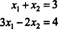## Introduction to Linear Systems

The basic problem of linear algebra is to solve a system of linear equations. A linear equation in the n variables—or unknowns— x 1, x 2, …, and x n is an equation of the formwhere b and the coefficients a i are constants. A finite collection of such linear equations is called a linear system. To solve a system means to find all values of the variables that satisfy all the equations in the system simultaneously. For example, consider the following system, which consists of two linear equations in two unknowns:Although there are infinitely many solutions to each equation separately, there is only one pair of numbers x 1 and x 2 which satisfies both equations at the same time. This ordered pair, (x 1, x 2) = (2, 1), is called the solution to the system.